Journal of Multimedia Information System
Korea Multimedia Society
Section C

# The Parameter Learning Method for Similar Image Rating Using Pulse Coupled Neural Network

Hiroki Matsushima1, Hiroaki Kurokawa1,*
1School of Engineering, Tokyo University of Technology, Tokyo, Japan, hkuro@cs.teu.ac.jp
*Corresponding Author: Hiroaki Kurokawa, 1404-1 Katakura Hachioji Tokyo Japan, +81-42-637-2404, hkuro@cs.teu.ac.jp.

© Copyright 2016 Korea Multimedia Society. This is an Open-Access article distributed under the terms of the Creative Commons Attribution Non-Commercial License (http://creativecommons.org/licenses/by-nc/4.0/) which permits unrestricted non-commercial use, distribution, and reproduction in any medium, provided the original work is properly cited.

Received: Dec 15, 2016 ; Revised: Dec 30, 2016 ; Accepted: Dec 31, 2016

Published Online: Dec 31, 2016

## Abstract

The Pulse Coupled Neural Network (PCNN) is a kind of neural network models that consists of spiking neurons and local connections. The PCNN was originally proposed as a model that can reproduce the synchronous phenomena of the neurons in the cat visual cortex. Recently, the PCNN has been applied to the various image processing applications, e.g., image segmentation, edge detection, pattern recognition, and so on. The method for the image matching using the PCNN had been proposed as one of the valuable applications of the PCNN. In this method, the Genetic Algorithm is applied to the PCNN parameter learning for the image matching. In this study, we propose the method of the similar image rating using the PCNN. In our method, the Genetic Algorithm based method is applied to the parameter learning of the PCNN. We show the performance of our method by simulations. From the simulation results, we evaluate the efficiency and the general versatility of our parameter learning method.

Keywords: Parameter learning; Pulse Coupled Neural Network; Similar image rating

## I. INTRODUCTION

Pulse-Coupled Neural Network (PCNN)  is a kind of neural network model composed of spiking neurons and local connections. The PCNN shows the temporary synchronous dynamics of neurons’ firings. By using the synchronous pulse dynamics of the PCNN, many engineering applications of the PCNN have been proposed especially in the fields of image processing, e.g., image segmentation, edge detection, pattern recognition, image matching and so on -, where the two-dimensional PCNN is used as shown in Fig.1.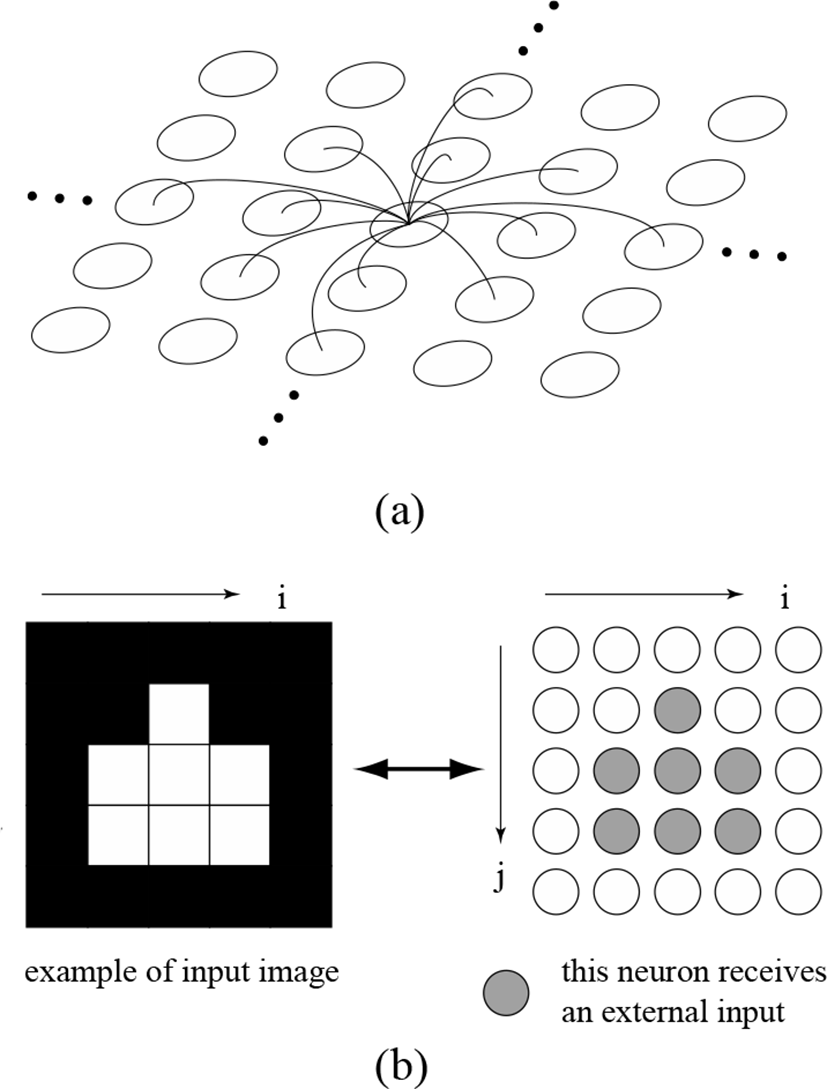Fig. 1. The basic scheme of the image processing using PCNN

The image matching method using the PCNN-Icon  is one of the valid applications of the PCNN. The PCNN-Icon is determined as the time series of the number of firing neurons. In this method the PCNN-Icon is used as an image feature amount, and a correlation coefficient of the PCNN-Icons form two images is used for the decision of their equality.

The PCNN has several parameters to be determined. It has been shown that the parameter learning based on the Genetic Algorithm  is effective in the method for the image matching.

In this study, we apply the two-dimensional PCNN to the similar image rating. In our method, the Genetic Algorithm based method is used for the parameter learning. We evaluate the effectiveness of the parameter learning method by simulations. The results suggest the possibility of application to the similar image search and the category classification of images.

## II. THE MODEL AND THE METHOD

2.1 Pulse Coupled Neural Network (PCNN)

Figure 1 shows the basic scheme of the image processing using two-dimensional PCNN. The spiking neurons in the PCNN are locally connected as shown in Fig. 1(a). The neuron in the PCNN and the pixel in the processing image are in one-to-one correspondence, and the intensity of the pixel can be neuronal external input as shown in Fig. 1(b).

The neuron in the PCNN is composed of three parts, i.e., the feeding input, the linking input, and the pulse generator. Figure 2 shows a simple schematic of the neuron in the PCNN.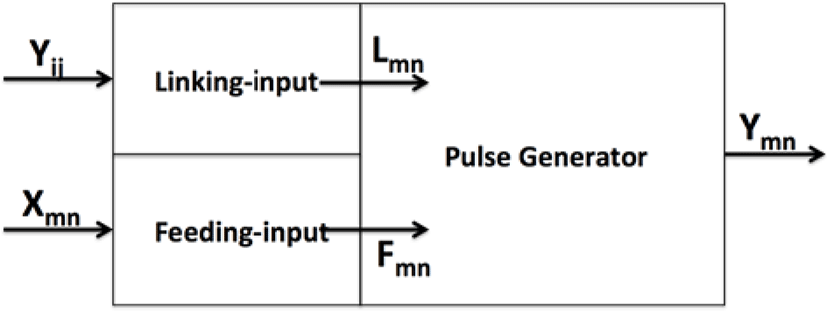Fig. 2. The schematic of the neuron Nmn in the PCNN

The Feeding input receives an external input such as an intensity of the corresponding pixel, and the linking input receives the inputs from other neurons. Assuming that the neurons Nmn arearranged in a two-dimensional lattice so as to correspond to the pixel Xmn of the image, the feeding input receives the external stimulus from the pixel Xmn, where m, n and i, j denote the coordinates of the neurons and pixels. Also the linking input receives the output of neighboring neurons as shown in Fig. 1(a).

In this study, we assume that the feeding input Fmn and linking-input Lmn at time step t are given by,

${F}_{mn}\left(t\right)={X}_{mn}}{255}$
(1)
${L}_{mn}\left(t\right)={L}_{mn}\left(t-1\right)exp\left(-1}{{\tau }_{L}}\right)\text{\hspace{0.17em}}\text{\hspace{0.17em}}+{V}_{L}\sum _{i}\sum _{j}{W}_{mn,ij}{Y}_{ij}\left(t-1\right),$
(2)

where τL and VL are the parameters. The feeding input is a normalized intensity of the corresponding pixel. The linking input is updated with the neighboring neurons’ outputs. Synaptic weights Wmn,ij of the neuron Nmn and neighboring neuron Nij is given by,

${W}_{mn,ij}\left(t\right)=\left\{\begin{array}{cc}1}{hd}& \left(r\ge d\right),\\ 0& otherwise.\end{array}$
(3)

In Eq. (3), d is given by,

$d=\sqrt{{\left(i-m\right)}^{2}+{\left(j-n\right)}^{2}}.$
(4)

In Eq. (3) and (4), r and h are the parameters. Note that the neuron has no synaptic weight to itself.

The pulse generator calculates the internal state of the neuron. The internal state of the neuron Nmn at time step t is given by,

${U}_{mn}\left(t\right)={F}_{mn}\left(t\right)\left(1+\beta {L}_{mn}\left(t\right)\right),$
(5)

where β is the parameter. When the internal state Umn exceeds the threshold Tmn, neurons Nmn fires. Firing condition of the neuron Nmn at the time step t is given by,

${Y}_{mn}\left(t\right)=\left\{\begin{array}{cc}1& {U}_{mn}\left(t\right)>{T}_{mn}\left(t\right),\\ 0& otherwise.\end{array}$
(6)

In Eq. 6, Tmn is the threshold and it is given by,

${T}_{mn}\left(t+1\right)={T}_{mn}\left(t\right)exp\left(-1}{{\tau }_{T}}\right)+{V}_{T}{Y}_{mn}\left(t\right),$
(7)

where τT and VT are the parameters. The firing state of the neuron is obtained by calculation of Eqs. (1) to (7). The firing states of the neurons are synchronously updated at each time step. The time series of the number of firing neurons in the PCNN is called the PCNN-Icon. Figure3 shows examples of PCNN-Icons.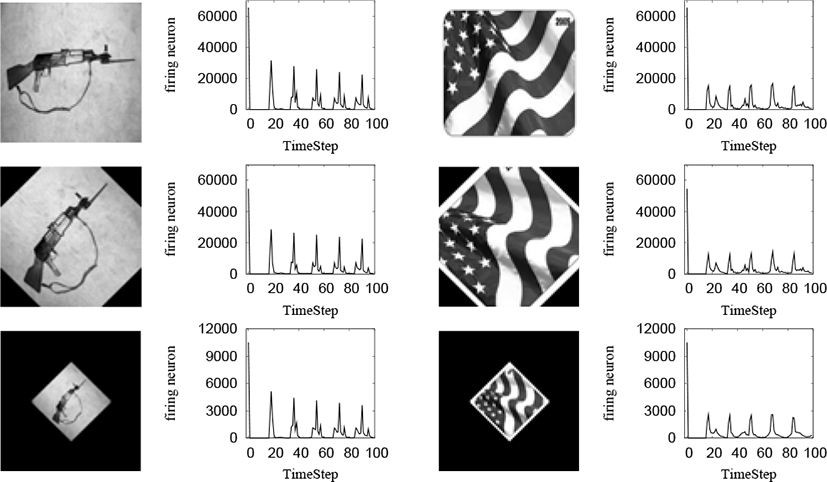Fig. 3. Example of PCNN-Icon

In our previous study , these PCNN-Icons are used as an image feature amount for the image matching. As shown in Figure 3, similar PCNN-Icons are obtained from same images, even if, the image is rotated or reduced.

The parameters of the PCNN can be summarized to a set of seven parameters in Eqs. (1) to (7). The performance of the application depends on these parameters. In this study, we apply the parameter learning method based on the Genetic Algorithm to obtain appropriate values of these seven parameters.

2.2 Parameter Learning Method

In this section, we describe the PCNN parameter learning method using the Genetic Algorithm. The Genetic Algorithm is adaptive heuristic search algorithm based on the evolutionary ideas from a natural selection and an alternation of generations.

In our previous study , it has been proposed that the Genetic Algorithm based method of the PCNN parameter learning for the image matching. In this study, we apply the similar method to the similar image rating.

To apply the Genetic Algorithm to the parameters optimization, a set of real numbers of the seven PCNN parameters is defined as a chromosome. The procedure of the Genetic Algorithm is summarized as follows.

Step 1: Initialize the population

All of the chromosomes in the population are initialized randomly.

Step 2: Fitness Function

Calculate the fitness of each chromosome. If the fitness satisfies the condition, the parameters are obtained. In other case, go to step 3.

Step 3: Best Chromosome Selection

Select the best fitness chromosome, and send it to the next generation.

Step 4: Crossover and Mutation

The remaining chromosomes are modified by Crossover and Mutation. Modified chromosomes are sent to the next generation. Repeat this step until a number of the next generation chromosomes are full, then return to the step 2.

Figure4 shows a flow diagram of this procedure. In our method, the seven learning PCNN parameters are β, τL, VL, τT, VT, h, and r in Eqs. (1) to (7). In our method, the number of chromosomes is 17, the crossover rate is 0.6, mutation rate is 0.3, and elitism and roulette selection are applied. The ranges of the real numbers are empirically assumed to be [0.0, 20.48]. Stop condition of the Genetic Algorithm is 1,000 iterations. The fitness is determined by the correlation coefficient CXY (0 < CXY <1) of the PCNN-Icons from image X and Y. The correlation coefficient is given by,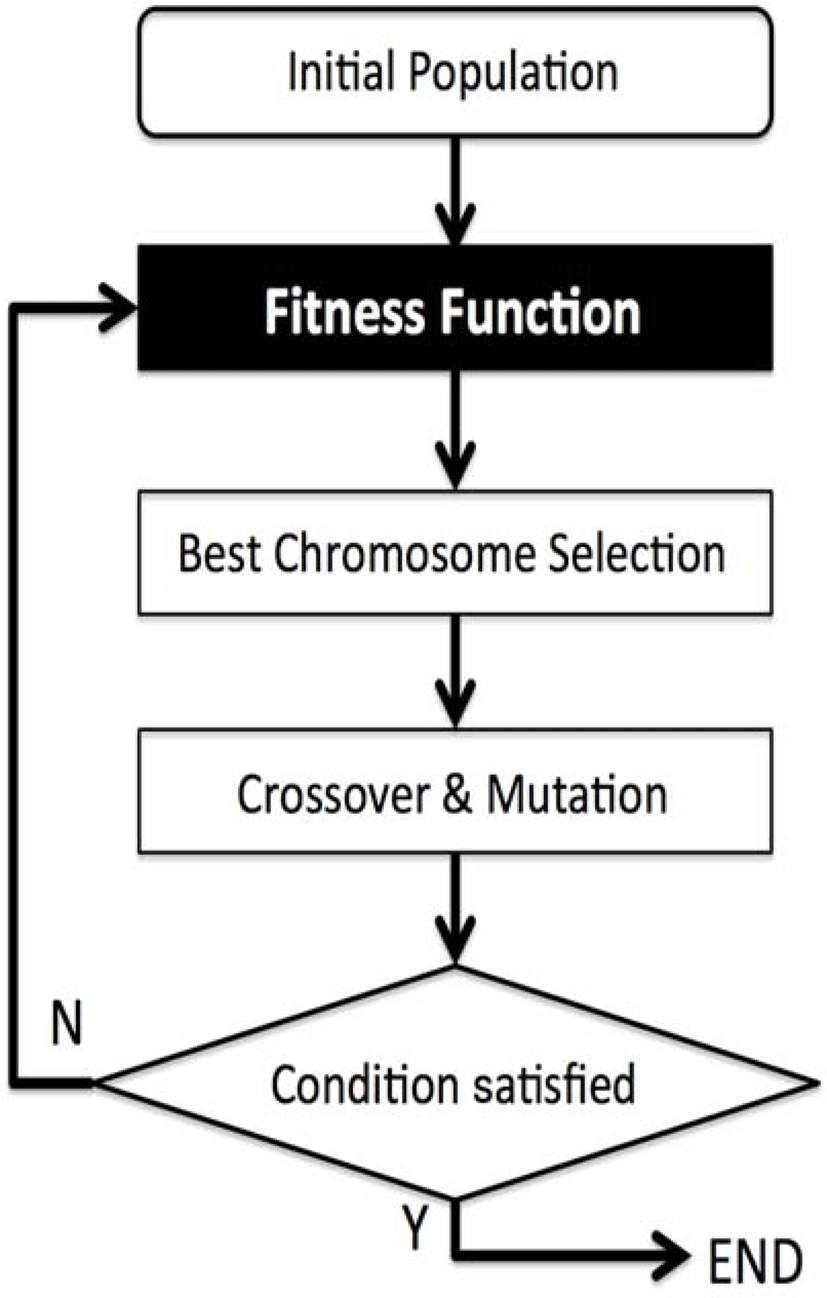Fig. 4. Procedure of the Genetic Algorithm for the parameter learning
${C}_{XY}=\frac{{\sum }_{t=0}^{{t}_{max}}\left({x}_{t}-\overline{x}\right)\left({y}_{t}-\overline{y}\right)}{\sqrt{{\sum }_{t=0}^{{t}_{max}}{\left({x}_{t}-\overline{x}\right)}^{2}}\sqrt{{\sum }_{t=0}^{{t}_{max}}{\left({y}_{t}-\overline{y}\right)}^{2}}}.$
(8)

As shown in Eq. (8), CXY is the normalized correlation coefficient, where xt and yt are the number of the firing neurons at the time step t. Also in Eq. (8), and are the average of xt and yt through t = 0 to t = tmax. We assume that the tmax is 100 in this study. The correlation range is normalized to [0.0, 1.0]. Also, the fitness is given by,

$Fitness=\sum {C}_{eq}+\sum \left(1-{C}_{diff}\right).$
(9)

In Eq. (9), Σ Ceq means the summation of correlation coefficients from the pairs of similar images, Σ( 1 − Cdiff ) means the summation of the values of formula including the correlation coefficients from the pairs of non-similar images. Figure 5 shows examples of similar images and non-similar images.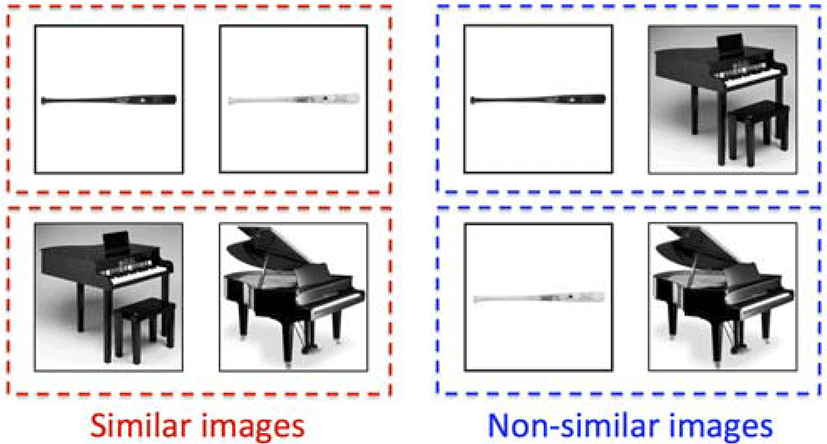Fig. 5. Similar images and non-similar images

## III. SIMULATION RESULTS

In this section, we show the simulation results of the similar image rating by our method. Figure 6 shows 18 test images used in the parameter learning. The set of these test images is composed of three similar image groups i.e., the baseball bat, the piano, and the motorcycle. The goal of this simulation is to find the similarity of the images in the same group by similar image rating.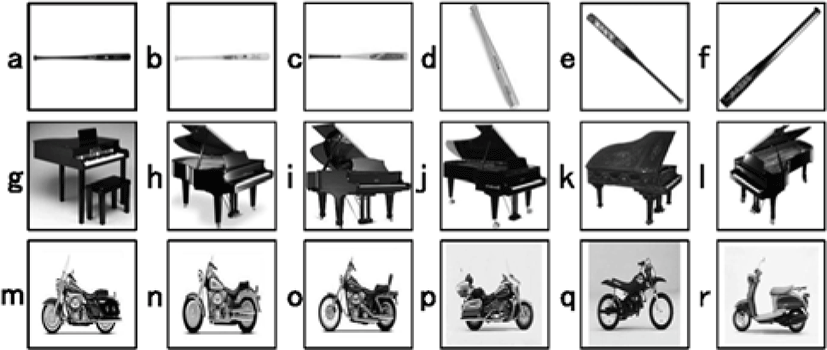Fig. 6. Test Images for the parameter learning

Figure 7 shows the results of the similar image rating by the PCNN with random parameters. The value in each cell shows the correlation coefficient of the PCNN-icons from the 18 test images. Also, Figure 8 is the results from the PCNN with parameters obtained by our method. In these results, the colored cells show the top six of large correlation coefficient. From these results, we can find that our method can decide the appropriate parameters for the similar image rating.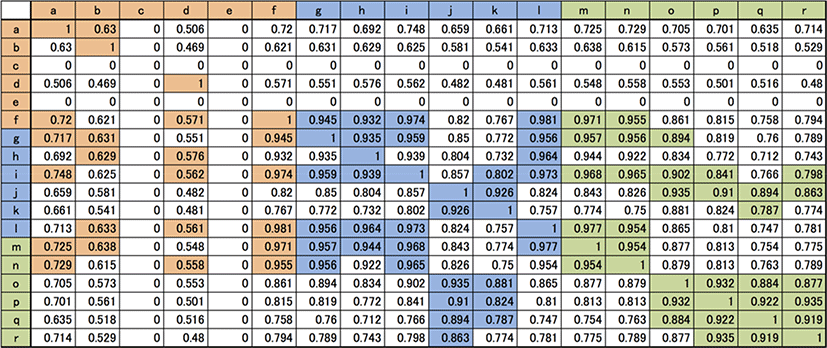Fig. 7. The results with the random parameters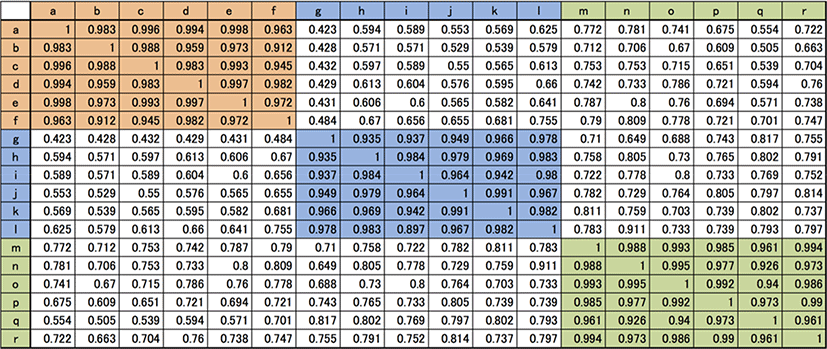Fig. 8. The results with the parameters by our method

In order to show the general versatility of our parameter learning method, we show the results of similar image rating using unlearned images. Figure 9 shows nine test images for the simulation. Figure 10 shows the results of the similar image rating by the PCNN with learned parameters using the images shown in Figure 6. From the results, we can find that our method has general versatility for the similar image rating.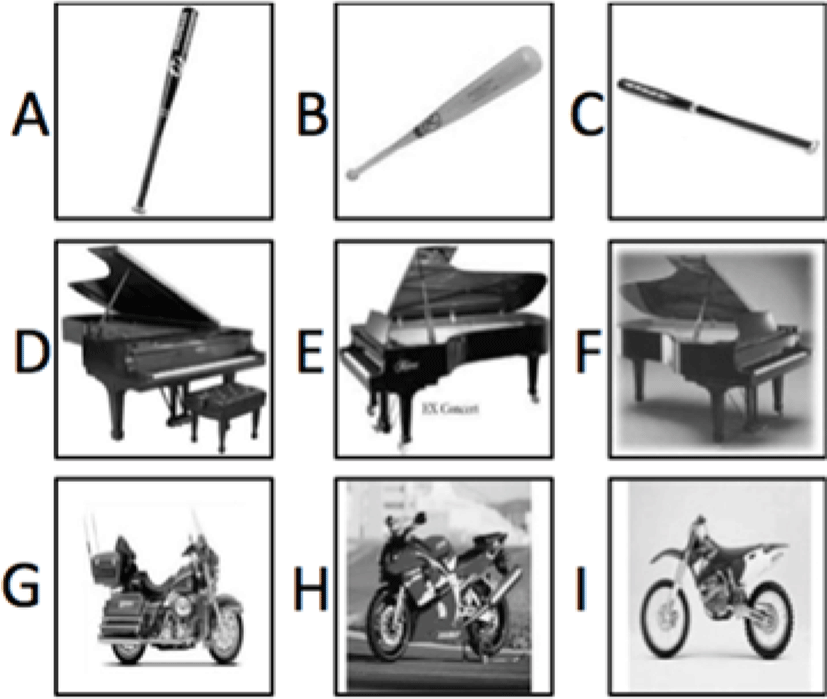Fig. 9. Test images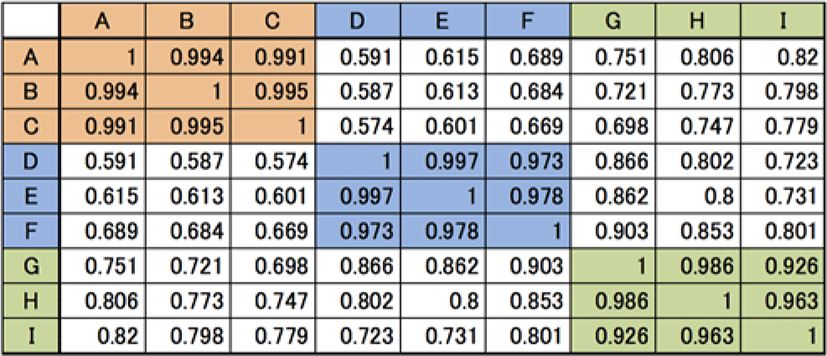Fig. 10. The results using test images

## IV. CONCLUSION

In this study, we applied our Genetic Algorithm based PCNN parameter learning method to the similar image rating using the PCNN, and evaluate its performances by the simulation. The simulation results showed that our method can find similarity among the same group images, and it is applicable not only the learned images but also the unlearned images.

The technique of the similar image rating can be a component technology of the Content-Based Image Retrieval (CBIR). We will apply our method to the CBIR system and show its efficiency in our future work.

## REFERENCES

.

R. Eckhorn, H. J. Reitboeck, M. Arndt, and P. Dicke, “Feature linking via synchronization among distributed assemblies,” Neural Computation, Vol.2, pp.293-307,1990..

R. Eckhorn, “Neural Mechanisms of Scene Segmentation: Recording from the Visual Cortex Suggest Basic Circuits for Linking Field Model,” IEEE Trans. Neural Network, vol.10, no.3, pp.464-479, 1999..

J.L. Johnson and M.L. Padgett, “PCNN Models and Applications,” IEEE Transactions on Neural Network, vol. 10, no. 3, pp.480-498, 1999..

H.S. Ranganth and G. Kuntimad, “Image segmentation using pulse coupled neural networks,” in Proceedings of the international Conference on Neural Networks, Orlando, vol. 2, 1285–1290, 1994..

T. Lindblad and J. M. Kinser, Image processing using pulse-coupled neural networks, Springer-Verlag, 2005..

H. Kurokawa, S. Kaneko, and M. Yonekawa, “A Color Image Segmentation using Inhibitory Connected Pulse Coupled Neural Network” in Proceedings of the international conference on Artificial neural network, Limassol, pp.776-783, 2009..

M. Yonekawa and H. Kurokawa, “The parameter optimization of the pulse coupled neural network for the pattern recognition,” in Proceedings of the international conference on Artificial neural network, Thessaloniki, pp.179-187, 2010..

M. Yonekawa and H. Kurokawa, “An evaluation of the image recognition method using pulse coupled neural network,” in Proceedings of the international conference on Artificial neural networks, Espoo, pp.217-224, 2011..

A.H.Weight, “Genetic algorithms for real parameter optimization,” Foundations of Genetic Algorithms, vol. 1, pp. 205-218, 1991.